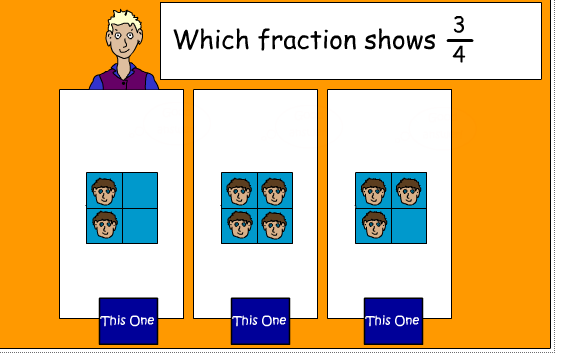## Wednesday, 19 September 2012

### MATHS FRACTIONS

In Maths this term we have been learning about FRACTIONSI can find fractions of whole numbers like:

½ of 12 = 6                   ⅓ of 9=  3              1/10 of 20= 2

⅔ of 12=  8                      ⅘ of 10=  8

I can recognise when a  fraction is greater than 1:

½ + ½ + ½ = 1 1/2

⅓ + ⅓ + ⅓ + ⅓ = 1 1/3

7/4 = 1 3/4